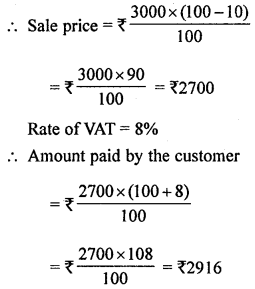## ML Aggarwal Class 8 Solutions for ICSE Maths Chapter 7 Percentage Check Your Progress

Question 1.
Convert $$\frac{7}{24}$$ into a percentage correct to foursignificant figures.
Solution:Question 2.
Express 750 mL as a percentage of 4 litres.
Solution:
Required Percentage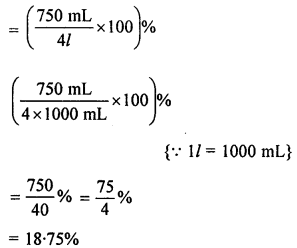Question 3.
A football team won 9 matches out of the total number of matches they played. If their win percentage was 45, then how many matches did they play in all?
Solution:
Let total matches = x
Matches won by a team = 9
Percentage = 45%
45% of x = 9
x= $$\frac{9 \times 100}{45}$$ =20
∴ No. of matches played = 20

Question 4.
In a straight student election, Rahul got 66% of the votes polled. If the defeated student got 187 votes, find the total number of votes polled.
Solution:
Rahul got votes in student election = 66%
Defeated student got votes = (100 – 66%) = 34%
Let the total number of votes = x
According to given,
34% of x = 187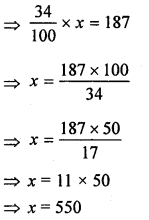Hence total number of votes = 550

Question 5.
36 kg of gunpowder contains 27 kg nitre, 5.4 kg charcoal and rest sulphur. Find the percentage content of sulphur in the gunpowder.
Solution:
Total mixture of gunpowder = 36 kg
Quantity of nitre = 27 kg
Quantity of charcoal = 5.4 kg
Rest quantity of sulphur = 36 – (27 + 5.4)kg
= 36 – 32.4 = 3.6 kg
∴ Percentage of sulphur = $$\frac{3.6 \times 100}{36}$$% = 10%

Question 6.
75% of the students in a class passed an exam. If 2 more students had passed the exam. 80% would have been successful. How many students are there in the class ?
Solution:
Let the total number of students in the class be x,
Number of passed students in the class = 75% of x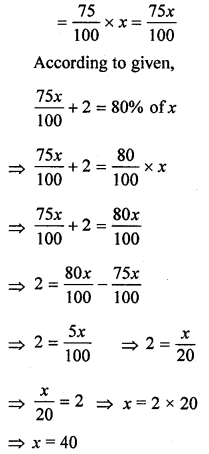Hence the total number of students in class = 40

Question 7.
The monthly salary of a school teacher in 2007 was ₹12000. It increased by 10% in 2008 and again by 10% in 2009. What is his salary in 2009?
Solution:
Total salary in 2007 = ₹ 12000
Increase in 2008 = 10%
and again increase in 2009 = 10%
∴ Salary in 2009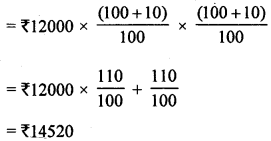Question 8.
Price of a commodity decreased by 10% last year and increased by 20% this year. Find the percentage change in two years.
Solution:
Let the Price of commodity = ₹ x
When the price of commodity decreased by 10% in last year
Then Price of a commodity in Last yearWhen the price of commodity increased by 20% in this year
Then price of commodity in this year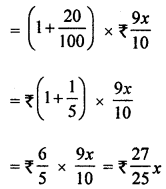Increased of price of commodity in these 2 years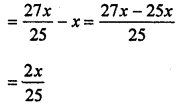% Increases of price of commodity in these 2 years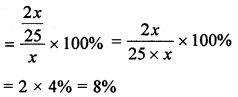Question 9.
A shop gives 15% discount. What would the sale price of each of these be?
(i) A dress marked at ₹150
(ii) A pair of shoes marked at ₹800.
Solution:
Rate of discount = 15%
(i) A dress marked at ₹ 150Question 10.
A refrigerator marked at ₹18000 is available for ₹17100. Find the discount given and the discount percent.
Solution:
Marked price of a regrigerator = ₹ 18000
Sale price = ₹17100
.∴ Discount = ₹18000 – ₹17100 = ₹900
Rate of discount =$$\frac{900 \times 100}{18000}$$ = 5%

Question 11.
Ramu purchased 20 parrots at ₹30 each. Two parrots flew away and Ramu sold the rest of the parrots at ₹40 each. Calculate the profit or loss percentage of Ramu.
Solution:
C.P. of one parrot = ₹30
C.P. of 20 parrots = ₹30 × 20 = ₹600
The Number of parrots flew away = 2
Number of rest of the parrots = 20 – 2 = 18
Then S.P. of remaining parrots = ₹40 × 18 = ₹ 720
Profit = S.P. – C.P. = ₹720 – ₹600 = ₹120Question 12.
Reena bought two tables for ₹ 1800 each. She sold one at a loss of 8% and the other at a profit of 12%. Find the selling price of each. Also, find out the total profit or loss.
Solution:
C.P. of one table = ₹1800
Loss = 8%Question 13.
Ajit bought an old car ₹78000. He spent ₹2000 on repairs and repainting the car. He sold the car to Anju at a gain of 15%. Anju sold it to Anthony at a loss of 5%. What did the car cost to Anthony?
Solution:
C.P. of car = ₹78000
Money spent on repairs and repainting ₹2000
Total C.P. of car = ₹78000 + ₹2000 = ₹80000
Ajit sold the car to Anju at a gain of 15%But this amount of car is C.P. for Anju.
∴ For Anju,
C.P. of Car = ₹92000
Loss = 5%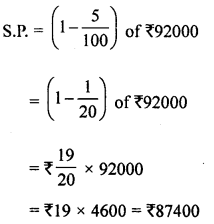Hence the car cost to Anthony = ₹87400

Question 14.
Gaurav sold an article at a profit of 12%. Had it been sold for ₹16 more, the profit would have been 20%. Find the C.P. of the article.
Solution:
Let the cost price of the article = ₹x,
Profit = 12%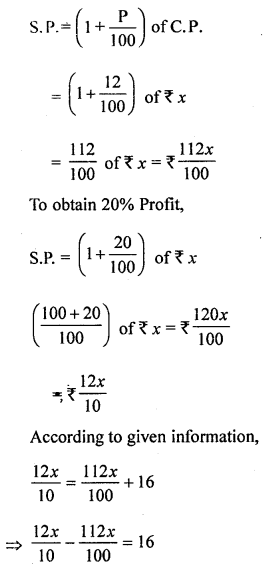Hence the cost price of the article = ₹200

Question 15.
A shopkeeper allows 8% discount on his goods and still earns a profit of 15%. If an article is sold for ₹460, find :
(i) the marked price
(ii) the cost price.
Solution:
(i) Since the shopkeeper allows a discount of 8%(ii) Let the cost price of the article be ₹x ;
Profit = 15%
Also, S.P. = ₹460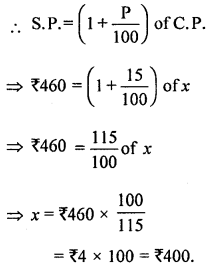Hence, the cost price of the article = ₹400

Question 16.
Rajesh bought an A.C. for ₹38500 including 10% VAT. Find the price ofA.C. before VAT was added.
Solution:
Total C.P. of an A.C. = ₹38500
VAT = 10%
Original price of AC = ₹ $$\frac{38500 \times 100}{100+10 \%}$$ = $$\frac{38500 \times 100}{110}$$ = ₹35000

Question 17.
A DVD player is marked for ₹3000. The shopkeeper offers 10% discount on it. If VAT is received 8%. Find the amount paid by the customer.
Solution:
Marked price of a DVD player = ₹3000
Discount = 10%